**

# 搜狗新闻语料文本分类实践

**

pip install jieba
pip install gensim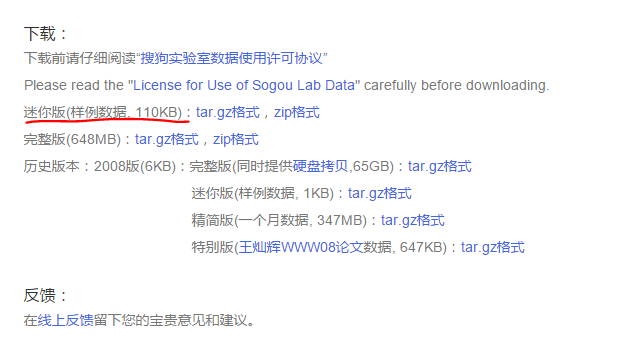<doc>
<url>http://gongyi.sohu.com/20120706/n347457739.shtml</url>
<contenttitle>深圳地铁将设立ＶＩＰ头等车厢　买双倍票可享坐票</contenttitle>
<content>南都讯　记者刘凡　周昌和　任笑一　继推出日票后，深圳今后将设地铁ＶＩＰ头等车厢，设坐票制。昨日，《南都ＭＥＴＲＯ》创刊仪式暨２０１２年深港地铁圈高峰论坛上透露，在未来的１１号线上将增加特色服务，满足不同消费层次的乘客的不同需求，如特设行李架的车厢和买双倍票可有座位坐的ＶＩＰ车厢等。论坛上，深圳市政府副秘书长、轨道交通建设办公室主任赵鹏林透露，地铁未来的方向将分等级，满足不同层次的人的需求，提供不同层次的有针对的服务。其中包括一些档次稍微高一些的服务。“我们要让公共交通也能满足档次稍高一些的服务”。比如，尝试有座位的地铁票服务。尤其是一些远道而来的乘客，通过提供坐票服务，让乘坐地铁也能享受到非常舒适的体验。他说，这种坐票的服务有望在地铁３期上实行，将加挂２节车厢以实施花钱可买座位的服务。“我们希望轨道交通和家里开的车一样，分很多种。”赵鹏林说，比如有些地铁是“观光线”，不仅沿途的风光非常好，还能凭一张票无数次上下，如同旅游时提供的“通票服务”。再比如，设立可以放大件行李的车厢，今后通过设专门可放大件行李的座位，避免像现在放行李不太方便的现象。“未来地铁初步不仅在干线上铺设，还会在支线、城际线上去建设。”“觉得如果车费不太贵的话，还是愿意考虑的。”昨日市民黄小姐表示，尤其是从老街到机场这一段，老街站每次上下客都很多人，而如果赶上上下班高峰期，特别拥挤，要一路从老街站站到机场，４０、５０分钟还是挺吃力的，宁愿多花点钱也能稍微舒适一点。但是白领林先生则表示，自己每天上下班都要坐地铁，出双倍车资买坐票费用有点高。</content>
</doc>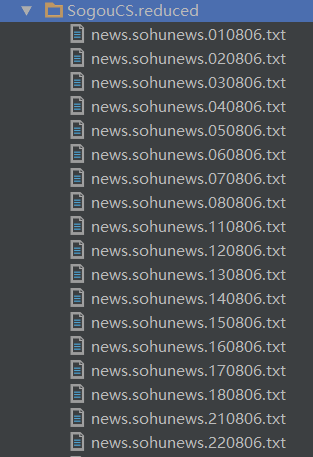<url>(.*?)</url> # 匹配URL
<content>(.*?)</content>  # 匹配content

SamplesGen.py

# -*- coding: utf-8 -*-
'''

content作为内容的txt文件存储
'''
import os
import re

'''生成原始语料文件夹下文件列表'''
def listdir(path, list_name):
for file in os.listdir(path):
file_path = os.path.join(path, file)
if os.path.isdir(file_path):
listdir(file_path, list_name)
else:
list_name.append(file_path)

'''字符数小于这个数目的content将不被保存'''
threh = 30
'''获取所有语料'''
list_name = []
listdir('SogouCS.reduced/',list_name)

'''对每个语料'''
for path in list_name:
print(path)

'''
正则匹配出url和content
'''
patternURL = re.compile(r'<url>(.*?)</url>', re.S)
patternCtt = re.compile(r'<content>(.*?)</content>', re.S)

classes = patternURL.findall(file)
contents = patternCtt.findall(file)

'''
# 把所有内容小于30字符的文本全部过滤掉
'''
for i in range(contents.__len__())[::-1]:
if len(contents[i]) < threh:
contents.pop(i)
classes.pop(i)

'''
把URL进一步提取出来，只提取出一级url作为类别
'''
for i in range(classes.__len__()):
patternClass = re.compile(r'http://(.*?)/',re.S)
classi = patternClass.findall(classes[i])
classes[i] = classi

'''
按照RUL作为类别保存到samples文件夹中
'''
for i in range(classes.__len__()):
file = 'samples/' + classes[i] + '.txt'
f = open(file,'a+',encoding='utf-8')
f.write(contents[i]+'\n')   #加\n换行显示


1. 该代码运行时仅能 decode UTF-8字符集，而搜狗语料库上下载的语料用记事本打开可以看出是ASCII编码方式。这里推荐可以用软件将多个语料文件转换编码为UTF-8方便今后处理。
2. 语料库文件打开后可以看到许多内容不全、内容过少甚至为空的语料，这部分语料通过一个threshold来控制，本文中采用30，即新闻内容少于50个字符时整个新闻内容和URL都不被记录为样本。
3. 另外内容不全的样本被剔除时不可简单地在for循环中使用pop()方法，因为 for i in range(len(vec)) 方法中使用pop方法时会导致数组 vec 的维度被减小，而 for 循环又不知道这个减小的事件，因此for还会尝试对len(vec) - 1等下标的访问，而此时该下标的数组元素已经不在，就会报出越界错误（别问我怎么知道的，写代码不小心真的要付出很大代价才能debug出来）。本文中采用for i in range(contents.len())[::-1]:从右向左取数即可。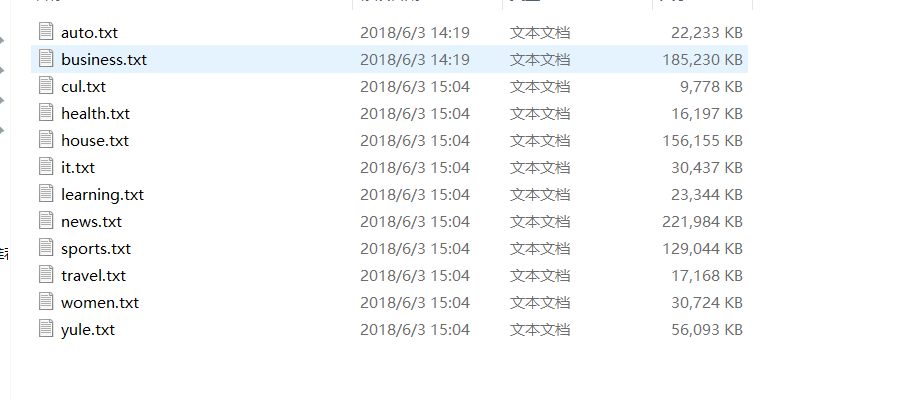（1）从samples文件夹中读取所有样本文件

textProcess.py (辅助函数保存脚本）

'''生成原始语料文件夹下文件列表'''
def listdir(path, list_name):
for file in os.listdir(path):
file_path = os.path.join(path, file)
if os.path.isdir(file_path):
listdir(file_path, list_name)
else:
list_name.append(file_path)

list_name = []
listdir('samples/', list_name)
for path in list_name[0:2]:
print(path)

class_count = 0
for text in file:
...

（2）分词

            content = text
# 分词
word_list = list(jieba.cut(content, cut_all=False))

（3）去停用词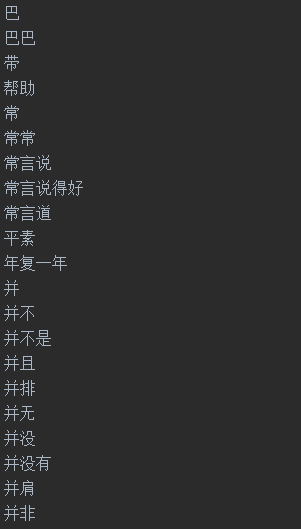def get_stop_words():
path = "stop_words"
return set(file)

def rm_stop_words(word_list):
word_list = list(word_list)
stop_words = get_stop_words()
# 这个很重要，注意每次pop之后总长度是变化的
for i in range(word_list.__len__())[::-1]:
# 去停用词
if word_list[i] in stop_words:
word_list.pop(i)
#  去数字
elif word_list[i].isdigit():
word_list.pop(i)
return word_list

def rm_word_freq_so_little(dictionary, freq_thred):
small_freq_ids = [tokenid for tokenid, docfreq in dictionary.dfs.items() if docfreq < freq_thred ]
dictionary.filter_tokens(small_freq_ids)
dictionary.compactify()

（4）统计词频，生成词袋

       for text in file:

# 打标签
class_count = class_count + 1

content = text
# 分词
word_list = list(jieba.cut(content, cut_all=False))
# 去停用词
word_list = rm_stop_words(word_list)

'''
转化成词袋
gensim包中的dic实际相当于一个map
doc2bow方法，对没有出现过的词语，在dic中增加该词语
如果dic中有该词语，则将该词语序号放到当前word_bow中并且统计该序号单词在该文本中出现了几次
'''
word_bow = dictionary.doc2bow(word_list)
bow.append(word_bow)

1. 单个词向量：( 5 , 2 )
5是该单词在dictionary中的序号为5，2是在这篇文章中出现了两次。
2. 一篇文章矩阵： [ (5 ,2) , (3 , 1) ]
在该文章中出现了5号单词两次，3号单词1次。

（5）生成TF-IDF矩阵
TF-IDF相比One-hot编码方式更加合理，它是根据该单词在当前文章中出现的频率和该单词在所有语料中出现的频率评估一个单词的重要性，当一个单词在这篇文章中出现的次数很多的时候，这个词语更加重要；但如果它在所有文章中出现的次数都很多，那么它就显得不那么重要。统计TF-IDF可以使用gensim提供的方法。

    # 去除过少单词 ps:可能导致维数不同
rm_word_freq_so_little(dictionary,freq_thred)

dictionary.save('dicsave.dict')
corpora.MmCorpus.serialize('bowsave.mm', bow)

tfidf_model = models.TfidfModel(corpus=bow,dictionary=dictionary)

with open('tfidf_model.pkl', 'wb') as f2:
pickle.dump(tfidf_model, f2)
'''训练tf-idf模型'''
corpus_tfidf = [tfidf_model[doc] for doc in bow]

'''将gensim格式稀疏矩阵转换成可以输入scikit-learn模型格式矩阵'''
data = []
rows = []
cols = []
line_count = 0
for line in corpus_tfidf:
for elem in line:
rows.append(line_count)
cols.append(elem)
data.append(elem)
line_count += 1

print(line_count)
tfidf_matrix = csr_matrix((data,(rows,cols))).toarray()

count = 0
for ele in tfidf_matrix:
# print(ele)
# print(count)
count = count + 1

    dictionary.save('dicsave.dict')
corpora.MmCorpus.serialize('bowsave.mm', bow)

（6）输入模型训练/预测

[ 0 , 0, 0, …, 0, 1 , 1 , … , 1]样本向量，其中0有100个，1有100个。

    # cut label 1 mil label 0
'''生成labels'''
labels = np.zeros(sum(labels_count) + 1)
for i in range(labels_count):
labels[i] = 1

    '''分割训练集和测试集'''
rarray=np.random.random(size=line_count)
x_train = []
y_train = []
x_test = []
y_test = []

for i in range(line_count-1):
if rarray[i]<0.8:
x_train.append(tfidf_matrix[i,:])
y_train.append(labels[i])
else:
x_test.append(tfidf_matrix[i,:])
y_test.append(labels[i])

x_train,x_test,y_train,y_test = train_test_split(tfidf_matrix,labels,test_size=0.3,random_state=0)

H(x) = w x + b，计算结果输入softmax函数，将H映射到 0-1 的区间作为概率预测， 可以采用梯度下降算法进行模型训练。

    '''LR模型分类训练'''
classifier=LogisticRegression()

classifier.fit(x_train, y_train)
#
# with open('LR_model.pkl', 'wb') as f:
#     pickle.dump(classifier, f)

print(classification_report(y_test,classifier.predict(x_test)))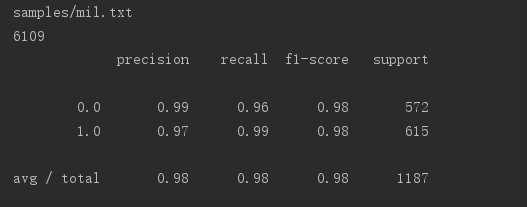textClsfy.py:

'''

'''
import numpy as np
from sklearn.linear_model.logistic import  *
from gensim import corpora, models, similarities
import jieba
from sklearn.model_selection import train_test_split
import pickle
from sklearn.discriminant_analysis import LinearDiscriminantAnalysis
from learnTextClsf.textProcess import *
from scipy.sparse import csr_matrix
from sklearn.metrics import classification_report

if __name__ == '__main__':
freq_thred = 10        # 当一个单词在所有语料中出现次数小于这个阈值，那么该词语不应被计入词典中

# 字典
dictionary = corpora.Dictionary()
# 词袋
bow  = []

labels_count = []
list_name = []
listdir('samples/', list_name)
count = 0

for path in list_name[0:2]:
print(path)

class_count = 0
for text in file:

# 打标签
class_count = class_count + 1

content = text
# 分词
word_list = list(jieba.cut(content, cut_all=False))
# 去停用词
word_list = rm_stop_words(word_list)

'''
转化成词袋
gensim包中的dic实际相当于一个map
doc2bow方法，对没有出现过的词语，在dic中增加该词语
如果dic中有该词语，则将该词语序号放到当前word_bow中并且统计该序号单词在该文本中出现了几次
'''
word_bow = dictionary.doc2bow(word_list)
bow.append(word_bow)

labels_count.append(class_count-1)

# with open('dictionary.pkl', 'wb') as f1:
#     pickle.dump(dictionary, f1)

# 去除过少单词 ps:可能导致维数不同
rm_word_freq_so_little(dictionary,freq_thred)

# dictionary.save('dicsave.dict')
# corpora.MmCorpus.serialize('bowsave.mm', bow)

tfidf_model = models.TfidfModel(corpus=bow,dictionary=dictionary)

# with open('tfidf_model.pkl', 'wb') as f2:
#     pickle.dump(tfidf_model, f2)
'''训练tf-idf模型'''
corpus_tfidf = [tfidf_model[doc] for doc in bow]

'''将gensim格式稀疏矩阵转换成可以输入scikit-learn模型格式矩阵'''
data = []
rows = []
cols = []
line_count = 0
for line in corpus_tfidf:
for elem in line:
rows.append(line_count)
cols.append(elem)
data.append(elem)
line_count += 1

print(line_count)
tfidf_matrix = csr_matrix((data,(rows,cols))).toarray()

count = 0
for ele in tfidf_matrix:
# print(ele)
# print(count)
count = count + 1

# cut label 1 mil label 0
'''生成labels'''
labels = np.zeros(sum(labels_count) + 1)
for i in range(labels_count):
labels[i] = 1

'''分割训练集和测试集'''
rarray=np.random.random(size=line_count)
x_train = []
y_train = []
x_test = []
y_test = []

for i in range(line_count-1):
if rarray[i]<0.8:
x_train.append(tfidf_matrix[i,:])
y_train.append(labels[i])
else:
x_test.append(tfidf_matrix[i,:])
y_test.append(labels[i])

# x_train,x_test,y_train,y_test = train_test_split(tfidf_matrix,labels,test_size=0.3,random_state=0)

'''LR模型分类训练'''
classifier=LogisticRegression()

classifier.fit(x_train, y_train)
#
# with open('LR_model.pkl', 'wb') as f:
#     pickle.dump(classifier, f)

print(classification_report(y_test,classifier.predict(x_test)))08-02
09-11168
03-063655
07-30
10-203358
05-195405
11-20
03-031万+
06-19
04-071903
03-134931
03-31
12-021万+
08-25261